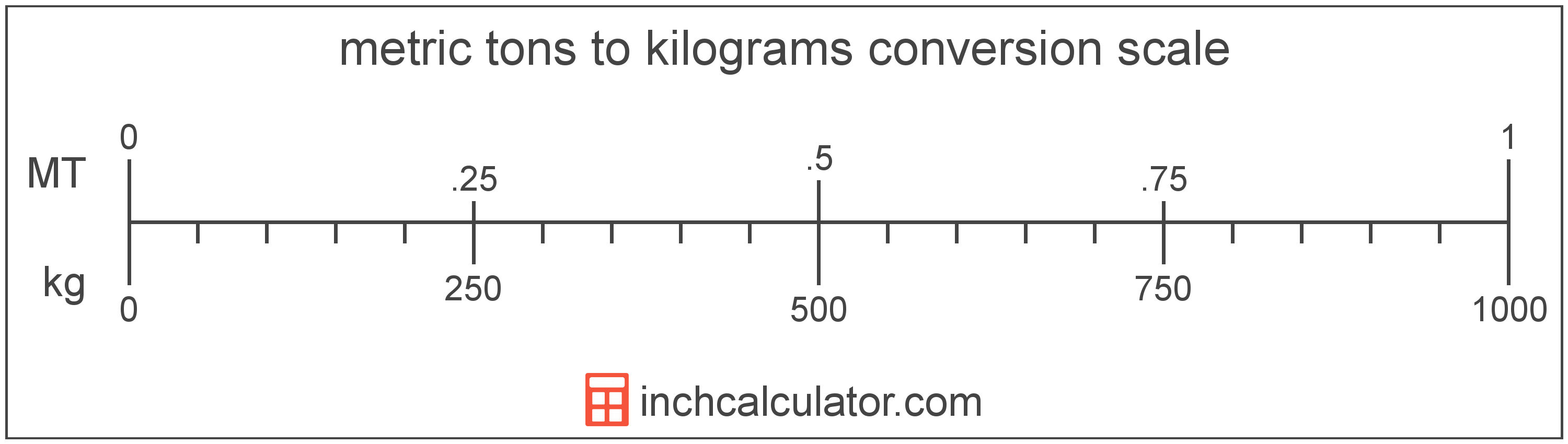# Metric Tons (Tonnes) to Kilograms Conversion

Enter the weight in metric tons below to get the value converted to kilograms.

Results in Kilograms:1 t = 1,000 kg

## How to Convert Metric Tons to KilogramsTo convert a metric ton measurement to a kilogram measurement, multiply the weight by the conversion ratio.

Since one metric ton is equal to 1,000 kilograms, you can use this simple formula to convert:

kilograms = metric tons × 1,000

The weight in kilograms is equal to the metric tons multiplied by 1,000.

For example, here's how to convert 5 metric tons to kilograms using the formula above.
5 t = (5 × 1,000) = 5,000 kg

### How Many Kilograms are in a Metric Ton?

There are 1,000 kilograms in a metric ton, which is why we use this value in the formula above.

1 t = 1,000 kg

## Metric Tons (Tonnes)

One tonne is equal to 1,000 kilograms or 2,204.6 pounds, and is used throughout the world as a unit of mass/weight. One tonne is also equal to 1 megagram. The metric ton should not be confused with short tons, used primarily in the US, or long tons, used primarily in the UK.

The metric ton is an SI accepted unit for weight for use with the metric system. A metric ton is sometimes also referred to as a tonne. Metric tons can be abbreviated as t, and are also sometimes abbreviated as T, Te, or MT. For example, 1 metric ton can be written as 1 t, 1 T, 1 Te, or 1 MT.

In the US the unit is referred to as the metric ton, while the rest of the world refers to it as the tonne. Most often it's still pronounced as tun, but it is sometimes pronounced tunny.

## Kilograms

The definition of the kilogram changed in 2019. One kilogram was previously equal to the mass of the platinum-iridium bar, known as the International Prototype of the Kilogram, which was stored in Sèvres, France.

The 2019 SI brochure now defines the kilogram using the Planck constant, and it is defined using the meter and second. It is roughly equal to the mass of 1,000 cubic centimeters of water.

The kilogram, or kilogramme, is the SI base unit for weight and is also a multiple of the gram. In the metric system, "kilo" is the prefix for 103. Kilograms can be abbreviated as kg; for example, 1 kilogram can be written as 1 kg.

## Metric Ton to Kilogram Conversion Table

Metric ton measurements converted to kilograms
Metric Tons Kilograms
0.001 t 1 kg
0.002 t 2 kg
0.003 t 3 kg
0.004 t 4 kg
0.005 t 5 kg
0.006 t 6 kg
0.007 t 7 kg
0.008 t 8 kg
0.009 t 9 kg
0.01 t 10 kg
0.02 t 20 kg
0.03 t 30 kg
0.04 t 40 kg
0.05 t 50 kg
0.06 t 60 kg
0.07 t 70 kg
0.08 t 80 kg
0.09 t 90 kg
0.1 t 100 kg
0.2 t 200 kg
0.3 t 300 kg
0.4 t 400 kg
0.5 t 500 kg
0.6 t 600 kg
0.7 t 700 kg
0.8 t 800 kg
0.9 t 900 kg
1 t 1,000 kg

## References

1. National Institute of Standards and Technology, Specifications, Tolerances, and Other Technical Requirements for Weighing and Measuring Devices, https://www.nist.gov/system/files/documents/2017/05/09/h44-01-all.pdf
2. International Bureau of Weights and Measures, The International System of Units, 9th Edition, 2019, https://www.bipm.org/documents/20126/41483022/SI-Brochure-9-EN.pdf